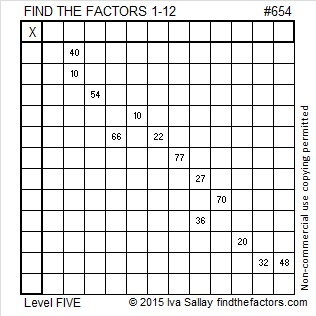# 654 and Level 5

654 is the sum of the fourteen prime numbers from 19 to 73.

654 is also the hypotenuse of the Pythagorean triple 360-546-654. Which of 654’s factors is the greatest common factor of those three numbers?Print the puzzles or type the solution on this excel file: 12 Factors 2015-10-19

—————————————————————————————————

• 654 is a composite number.
• Prime factorization: 654 = 2 x 3 x 109
• The exponents in the prime factorization are 1, 1, and 1. Adding one to each and multiplying we get (1 + 1)(1 + 1)(1 + 1) = 2 x 2 x 2 = 8. Therefore 654 has exactly 8 factors.
• Factors of 654: 1, 2, 3, 6, 109, 218, 327, 654
• Factor pairs: 654 = 1 x 654, 2 x 327, 3 x 218, or 6 x 109
• 654 has no square factors that allow its square root to be simplified. √654 ≈ 25.57342.—————————————————————————————————This site uses Akismet to reduce spam. Learn how your comment data is processed.